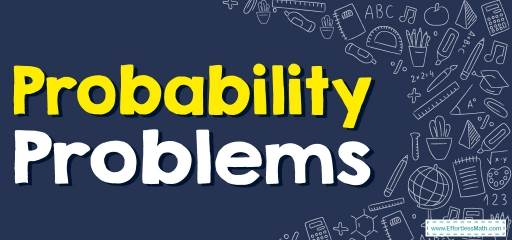# How to Solve Probability Problems? (+FREE Worksheet!)

Do you want to know how to solve Probability Problems? Here you learn how to solve probability word problems.## Step by step guide to solve Probability Problems

• Probability is the likelihood of something happening in the future. It is expressed as a number between zero (can never happen) to $$1$$ (will always happen).
• Probability can be expressed as a fraction, a decimal, or a percent.
• To solve a probability problem identify the event, find the number of outcomes of the event, then use probability law: $$\frac{number\ of \ favorable \ outcome}{total \ number \ of \ possible \ outcomes}$$

The Absolute Best Book to Ace Algebra I

### Probability Problems – Example 1:

If there are $$8$$ red balls and $$12$$ blue balls in a basket, what is the probability that John will pick out a red ball from the basket?

Solution:

There are $$8$$ red balls and $$20$$ a total number of balls. Therefore, the probability that John will pick out a red ball from the basket is $$8$$ out of $$20$$ or $$\frac{8}{8+12}=\frac{8}{20}=\frac{2}{5}$$.

### Probability Problems – Example 2:

A bag contains $$18$$ balls: two green, five black, eight blue, a brown, a red, and one white. If $$17$$ balls are removed from the bag at random, what is the probability that a brown ball has been removed?

Solution:

If $$17$$ balls are removed from the bag at random, there will be one ball in the bag.
The probability of choosing a brown ball is $$1$$ out of $$18$$. Therefore, the probability of not choosing a brown ball is $$17$$ out of $$18$$ and the probability of having not a brown ball after removing $$17$$ balls is the same.

## Exercises for Solving Probability Problems

The Absolute Best Book to Ace Pre-Algebra

### Solve.

1. A number is chosen at random from $$1$$ to $$10$$. Find the probability of selecting a $$4$$ or smaller.
2. A number is chosen at random from $$1$$ to $$50$$. Find the probability of selecting multiples of $$10$$.
3. A number is chosen at random from $$1$$ to $$10$$. Find the probability of selecting of $$4$$ and factors of $$6$$.
4. A number is chosen at random from $$1$$ to $$10$$. Find the probability of selecting a multiple of $$3$$.
5. A number is chosen at random from $$1$$ to $$50$$. Find the probability of selecting prime numbers.
6. A number is chosen at random from $$1$$ to $$25$$. Find the probability of not selecting a composite number.

1. $$\color{blue}{\frac{2}{5}}$$
2. $$\color{blue}{\frac{1}{10}}$$
3. $$\color{blue}{\frac{1}{2}}$$
4. $$\color{blue}{\frac{3}{10}}$$
5. $$\color{blue}{\frac{3}{10}}$$
6. $$\color{blue}{\frac{9}{25}}$$

The Best Books to Ace Algebra

### What people say about "How to Solve Probability Problems? (+FREE Worksheet!) - Effortless Math: We Help Students Learn to LOVE Mathematics"?

No one replied yet.

X
45% OFF

Limited time only!

Save Over 45%

SAVE $40 It was$89.99 now it is \$49.99